Breaking News
Home / Civil Engineering / Bar Bending Schedule / How To Calculate The Cutting Length Of Stirrups In Beam And Column

# How To Calculate The Cutting Length Of Stirrups In Beam And Column

### How To Calculate The Cutting Length Of Stirrups In Beam And Column

To cater the stresses and loads in RCC, Bars are bent to different shapes in the bar bending schedule.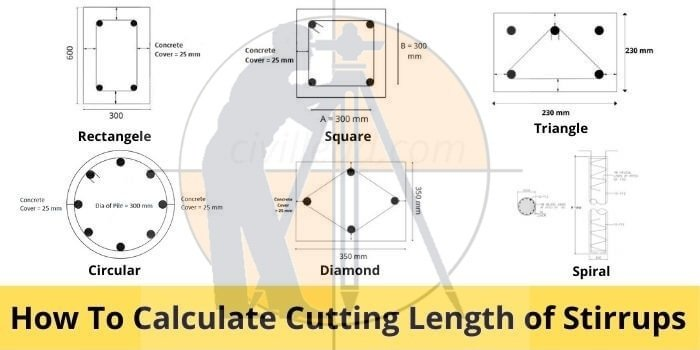Different shapes of bars have different cutting length. In this post, we are going to explain you about “How to calculate or find the cutting length of Stirrups for different shapes”.

Remember,

The transverse reinforcement provided in Column is called Ties and the transverse reinforcement provided in Beam is called Stirrups. But on-site, we usually call both transverse reinforcements as Stirrups.

The prime reason for providing the stirrups in the beam is for shear requirements and to keep the longitudinal bars in position. If you wanted to know more about the why stirrups & ties provided in the beam and column you can refer here

Deducting the concrete cover is most important in Bar bending, if you dont know how to deduct the concrete cover then refer this post

Concrete Cover deductions in Beams, Columns, Slab, Footings

# Steps involved in finding the cutting length of stirrups:-

1. Look at the size of column or beam from drawings
2. Adopt Dia of the bar (generally 8mm Dia is used for stirrups)
3. Deduct the concrete cover or clear cover
4. Find the total outer length of stirrup after deducting concrete cover.
5. Add the length of the hook to the length of the stirrup
6. Deduct the length of bends
7. Use below formula to find the total cutting length of stirrups

### How To Calculate The Quantity Of Bricks in 1 m3

Formula:

Cutting Length of Stirrups = Perimeter of Shape + Total hook length – Total Bend Length

Important Basic formulas:

Perimeter of Rectangle = 2 ( length + breadth)

Perimeter of Square = 4 x side length

Perimeter of circle or Circumference of Circle = 2πr = πd (r= radius, d= Diameter of Circle)

## Typical Diagram of Stirrup:-

Refer the below image of the typical diagram of stirrup for clear understanding about x & y length, bends, hooks and concrete cover.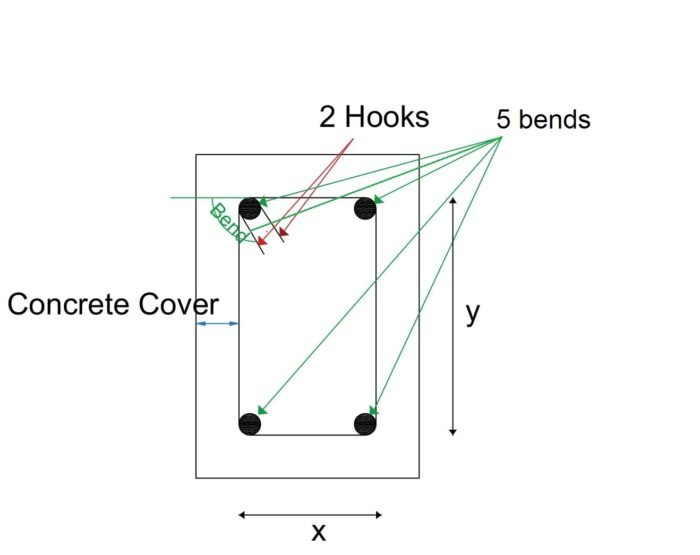In the above image, there are 5 bends at 4 corners, 2 hooks and concrete cover around the stirrup.
x = length of the stirrup in the x-direction after deducting concrete cover &
y = length of the stirrup in the y-direction after deducting concrete cover.

## Important standards used in Bends & Hooks:

The below standards are most important in calculating the hook length and bend lengths at corners while finding cutting length of stirrups.

• 1 Hook length = 9d or 75mm
• 45° Bend length = 1d
• 90° Bend length = 2d
• 135° Bend length = 3d

Remember, d = Diameter of Bar

## Cutting length for Rectangular Stirrups:-

Rectangular column or rectangular beam is the most commonly used shape of the column in any construction. In this shape of beam or column, a rectangular stirrup is usually adopted.

1. Considering the below Rectangular column size 230mm x 450mm for calculation purpose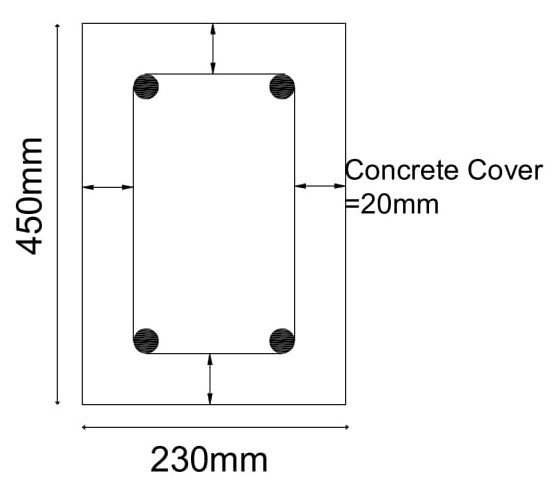1. Adopting Dia of Bar used for stirrups is 8mm
2. Deducting the concrete cover 20mm from all sides
x = 230 – 20-20 = 190mm
y = 450-20-20 = 410mm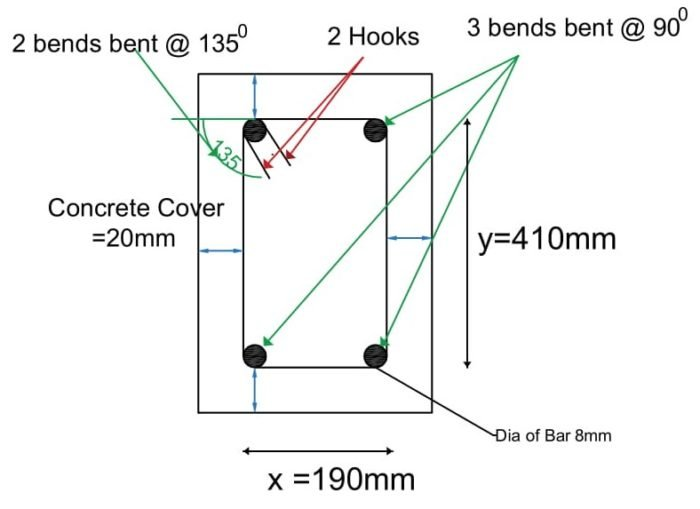1. Total Length of the hooks:
From fig, There are two hooks which means 9d+9d = 18d
2. Total Length of Bends:
From above fig, There are 3 bends which are bent at an angle of 900 and two bends are bent at an angle of 1350
Total bend length = 3 x 900 Bend length + 2 x 1350 Bend length = 3 x 2d + 2 x 3d = 12d = 12 x 8 = 96mm

Total Cutting length of Rectangular Stirrup = Perimeter of Rectangle + Total Hook length – Total Bend Length

= 2 (x+y) +18d – 12d = 2(190 + 410) + 18 x 8 – 12 x 8 = 1248mm = 1.248m

## Cutting length for Square Stirrups:-

1. Considered the column size as 450mm x 450mm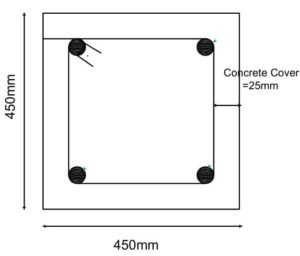1. Adopting Dia of Bar used for stirrups is 8mm
2. Deducting the concrete cover 25mm from all sides (in square all sides are equal)
x = 450- 20-20 = 410mm
y = 450-20-20 = 410mm, Hence x = y (in square all sides are equal)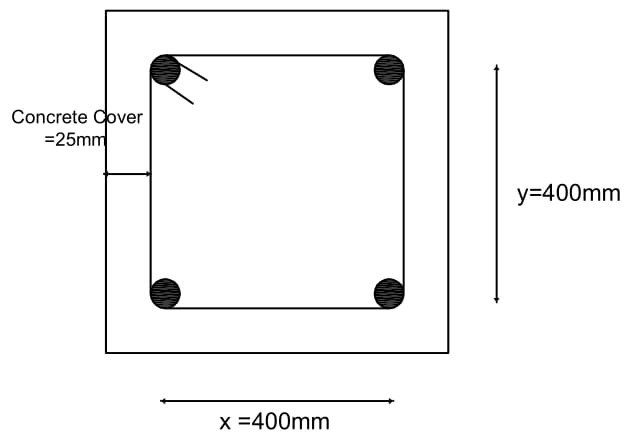1. Total Length of the hook:
There are two hooks which mean 9d+9d = 18d
2. Total length of Bends:
There are 3 bends which are bent at an angle of 900 and one is bent at an angle of 1350.
Total bend length = 3 x 900 Bend length + 2 x 1350 Bend length = 3 x 2d + 2 x 3d = 12d = 12 x 8 = 96mm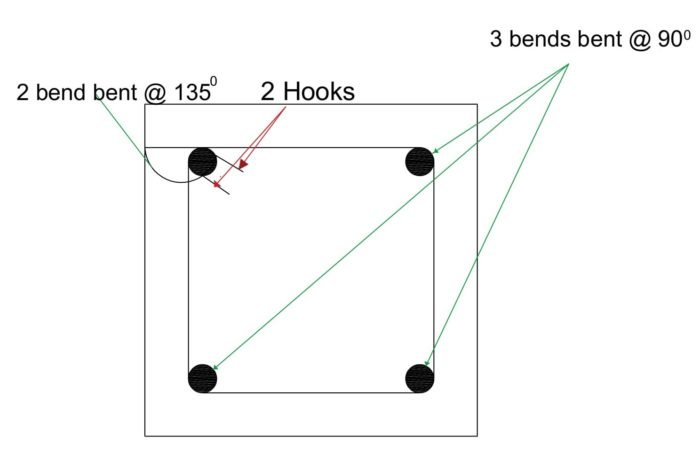Total Cutting length of Square Stirrup = Perimeter of Square + Total Hook length – Total Bend Length

= 4 x 410 +18d – 12d = 1648mm = 1.64m

## Cutting Length for Circular Stirrup:

1. Considered the column dia as D = 1000mm
2. Adopting Dia of Bar used for stirrups is d =8mm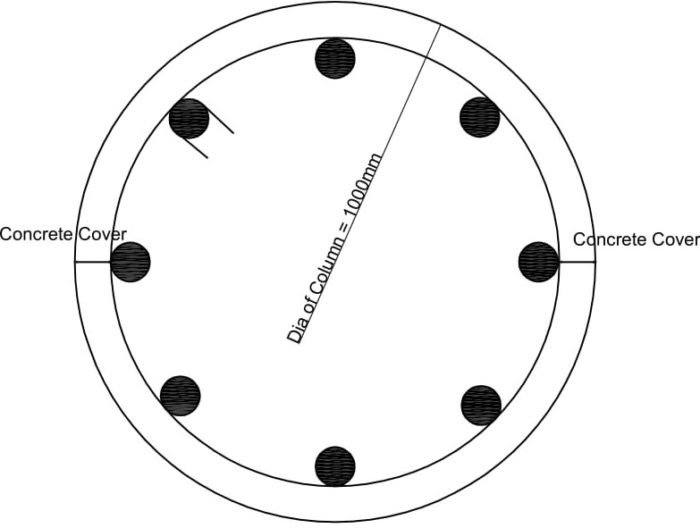Deducting the concrete cover from diameter of column
D = 1000-25-25 = 950mm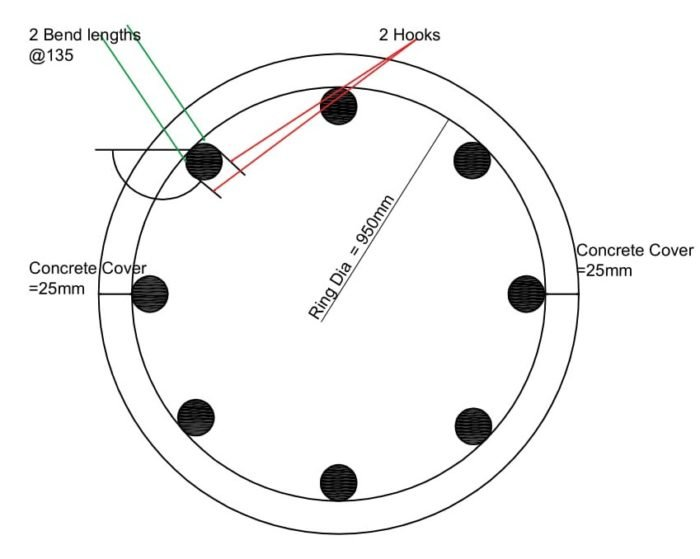1. Circumference length of Ring = πD = 950 x 3.14 = 2983mm
2. Total Length of the hook:
There are two hooks which means 9d+9d= 18d
3. Total Length of Bends:
There are 2 bends which are bent at an angle of 1350
Total bend length = 2 x 1350 Bend length = 2 x 3d = 6d= 6 x 8 = 48mm

Total Cutting length of Circular Stirrup or Ring = Circumference of Circle + Total Hook length – Total Bend Length
= 2983 +18d – 6d =3079mm =3.07m

## Cutting Length for Triangular Stirrups:

1. Considered the Column size 400mm x 450mm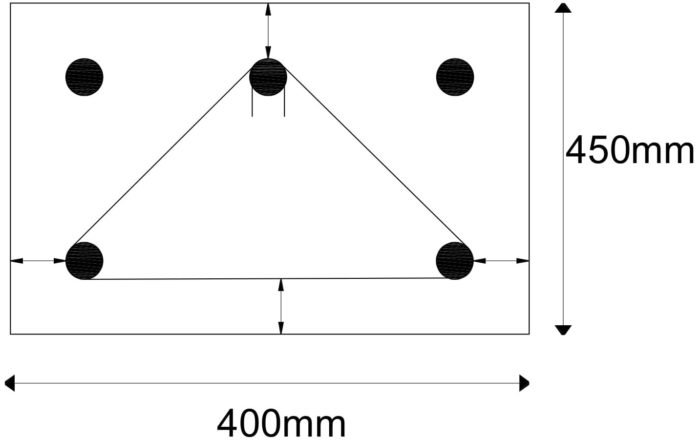1. Adopting Dia of Bar used for stirrups is d = 8mm
2. Deducting the concrete cover 25mm from all sides
x = 400-20-20 = 360mm
y = 450-20-20 = 410mm
From Pythagorean theorem,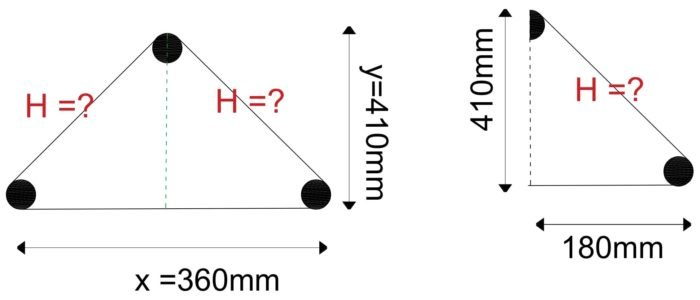1. look at 2nd triangle in above image
H2=(x/2)2 + y2
H2=1802 + 4102 => =  √(447)2  = 447mm
The total length of stirrup till now = 2 x H + 360 = 2 x 447 + 360 = 1254mm
2. Total Length of the hooks:
There are two hooks which means 9d+9d= 18d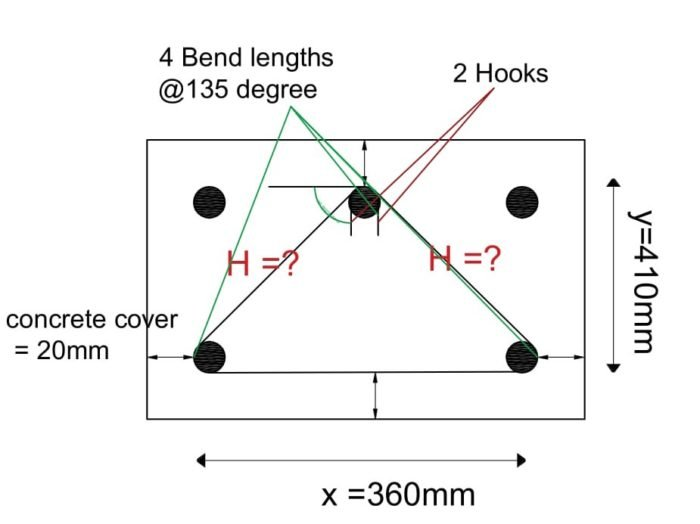1. Total length of Bends:
There are 4 bends which are bent at an angle of 1350
Total bend length =4 x 1350 Bend length=  4 x 3d = 12d= 12 x 8 = 96mm

Total Cutting length of Triangular Stirrup = Perimeter of Triangle + Total Hook length – Total Bend Length

= 1254+18d – 12d = 1302mm = 1.3m

## Cutting Length for Diamond Stirrups:

1. Considered the Column size 400mm x 400mm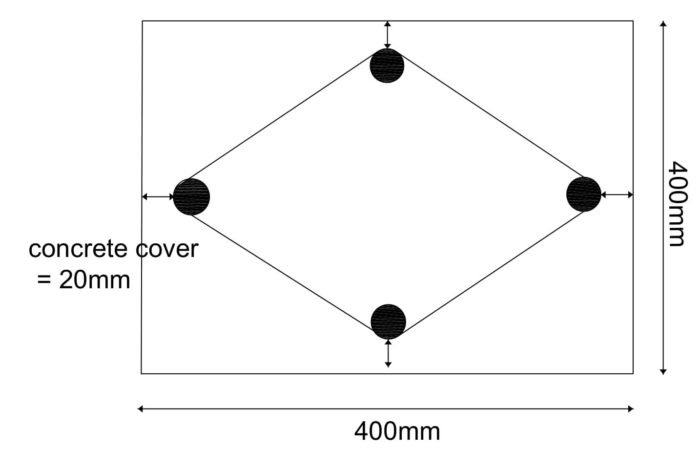1. Adopting Dia of Bar used for stirrups is d = 8mm
2. Deducting the concrete cover 25mm from all sides
x = 400-20-20 = 360mm
y = 400-20-20 =360mm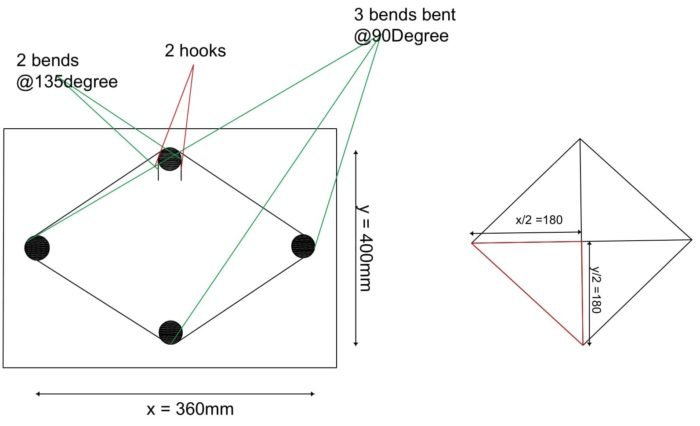1. From Pythagorean theorem,
H2=(x/2)2 +( y/2)2
H2=1802 + 1802 => =  √(254)2  = 254mm
2. The total length of stirrup = 4 x H  =4 x 254 = 1016mm
3. Total Length of the hook:
There are two hooks which means 9d+9d= 18d
4. Total length of Bends:
There are 3 bends which are bent at an angle of 90 + 2 bends which are bent at an angle of 1350
Total bend length = 3 x 900 Bend length + 2 x 1350 Bend length= 3 x 2d + 2 x 3d = 12d = 12 x 8 = 96mm

Total Cutting length of Diamond  Stirrup = Perimeter of Diamond shape + Total Hook length – Total Bend Length
= 1016+144-96 = 1064mm = 1.064mm

### THANKS.